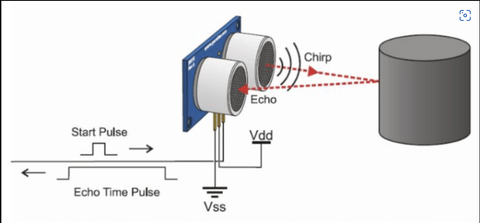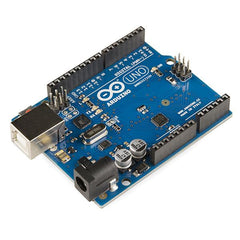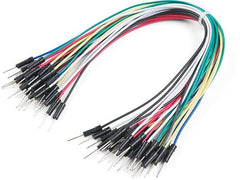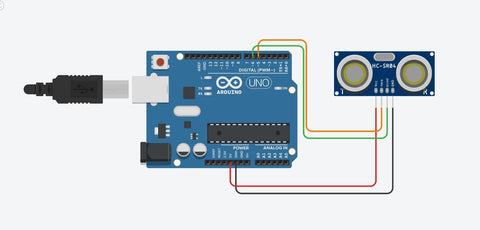# Speed Measurement using HC-SR04 Ultrasonic Sensor

Do you want to learn how to accurately measure speed using an affordable and easy-to-use ultrasonic sensor? If the answer is Yes! then check out this blog post which talks about Speed Measurement using HC-SR04 Ultrasonic Sensor. The article discusses the workings of HC-SR04, a commonly used ultrasonic sensor, and the basic principles of speed measurement. It also provides step-by-step instructions on how to build a speed measurement system using HC-SR04 and Arduino.

In this tutorial, we will be learning how to make a simple speed measurement device using the HC-SR04 Ultrasonic sensor and Arduino

## What is an Ultrasonic Sensor (HC-SR04)?

Ultrasonic sensor is a sensor that accurately measure distance using ultrasonic waves. It a non-contact sensor used to accurately measure distance between the sensor and the object ranging from 4 centimeters to 4 meters. It is quite inexpensive and widely available, making it suitable for all kinds of DIY projects.

The ultrasonic sensor calculates the distance by recording the time take for the emitted ultrasonic wave to reflect back from the object.

The sensor first sends an ultrasonic pulse and sees how long it takes for the receiver to receive back the emitted pulse. Since we know the speed of sound, we can use simple speed formulae to calculate the distance that the sound wave might have traveled. Additionally, we will divide the result by 2 since the sound would have travelled twice the distance between sensor and the object – from sensor to object and back.The HC-SR04 consists of four pins, namely

• VCC (5V Power)
• Trig (Trigger)
• GND (Ground)

VCC and GND pins will be used to power the device while Trigger pin needs to be configured as an digital output pin while the echo pin needs to be declared as digital input pin.

The above pins can be connected to any microcontrollers, but for this guide we will be using the Arduino

## Components Required to Build a Speed Measuring Device are:

1. Arduino Uno (any Arduino can be used)## Software Requirement

We will be using Arduino IDE to program our Arduino to read values from the ultrasonic sensor.

Alternatively, other popular code editors like PlatfromIO in Visual studio code can also be used but for simplicity we will be sticking with Arduino IDE

## Working Concept of Speed Measuring Device

To calculate the speed, the ultrasonic sensor needs to read the distance of object at least twice between a fixed interval of time, say 1s.

Let’s visualize this with an example,

Object X is moving with speed Y

The ultrasonic sensor is will measure the distance of the object every 1 second.

When the sensor measures the distance of the object the 1st time, the object X is at position A

When the sensor measures the distance of the object the 2nd time, the object X is at position B

Since the object moved |A-B| distance in 1 second, using the speed formulae, we can calculate the speed of the object

speed = distance / time

Here, speed = |A-B| / 1

The given result will be in the units of cm per second or cm/s

## Circuit DiagramThe ultrasonic and Arduino connections are shown above as follows

 Arduino Ultrasonic Sensor 5V VCC GND GND Pin 5 TRIG Pin 6 ECHO

## Code

``````
// Declaration of trigger and echo pins

int trigPin = 5;

int echoPin = 6;

// declaration of variables going to be used for the program

int distance1 = 0;

int distance2 = 0;

int measured_speed = 0;

long duration = 0;

int distance = 0;

void setup()

{

// Setting trigger pin as OUTPUT

pinMode(trigPin, OUTPUT);

// Setting echoPin as INPUT

pinMode(echoPin, INPUT);

// Starts the serial communication at baud rate 9600

Serial.begin(9600);

}

void loop()

{

// Measuring distance 1

delay(1000);                  // gives delay of 1 second

// Formula to calculate speed from distance1 and distance2

// We are dividing it by 1, since the time interval between the two distance measurement is 1000 ms or 1 second

measured_speed = abs(distance2 - distance1) / 1.0;

// Displaying the speed value on the serial monitor

Serial.print("Speed in cm/s :");

Serial.println(measured_speed);

}

// Function declaration to measure the distance based on the working principle of ultrasonic sensor

{

// Sets the trigPin on HIGH state for 10 micro seconds

digitalWrite(trigPin, HIGH);

delayMicroseconds(10);

digitalWrite(trigPin, LOW);

// Waits for the amount of time echoPin remains high and records the duration of the same

duration = pulseIn(echoPin, HIGH);

// Calculates the distance based on the speed of sound in ambient air

// and divide it by two since the sound traveled twice - once to the object and then back

distance = duration * 0.034 / 2;

// returning measured distance

return distance;

}
``````

## Conclusion

In this blog post, we have learned Speed Measurement using HC-SR04 Ultrasonic Sensor. By accurately calculating distance and time, this compact sensor can help you track the speed of moving objects with precision and ease. Whether you're a hobbyist or a tech enthusiast, the HC-SR04 is a versatile tool that can bring your projects to the next level. So what are you waiting for? Start exploring the endless possibilities of speed measurement today, and see how the HC-SR04 can help you achieve your goals faster than ever before!

If you appreciate our work don't forget to share this post and leave your opinion in the comment box.

Please do check out other blog posts about Interfacing ACS712 with Arduino , Arduino Interfacing with Ultrasonic Sensor , LED Interfacing with Arduino , Interfacing GSM Module with Arduino , Interfacing MAX30100 Pulse Oximeter with Arduino , IR Sensor Interfacing with Arduino , How to connect ZMPT101B to Arduino and  How to use Buzzer with Arduino.

Make sure you check out our wide range of products and collections (we offer some exciting deals!)

### 1. Can ultrasonic sensor measure speed?

Ultrasonic sensors can actually figure out how fast an object is going by seeing how long it takes to move a certain distance in front of the sensor. Then, they just use some formula to calculate the speed based on the distance and time. But here's the thing - it only works if the object is moving in a straight line and keeping a pretty consistent speed.

### 2. Can ultrasonic sensor detect motion?

Ultrasonic sensors can totally detect motion! They work by sending out super high-pitched sound waves and then measuring how long it takes for those waves to bounce back off an object. By analyzing the changes in the way the sound waves reflect, the sensor can pick up on any movement happening within its detection range. It's pretty sweet, and you can use these sensors for all kinds of cool stuff like security systems, robots, and motion detectors.